## Tamilnadu Samacheer Kalvi 6th Maths Solutions Term 3 Chapter 3 Perimeter and Area Intext Questions

Activity (Text book Page No. 39)

Question 1.
Observe the following shapes and answer the questions given below.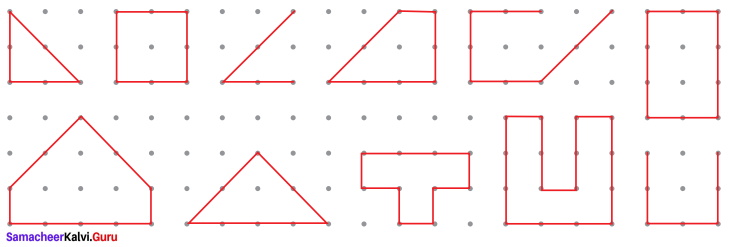i) Mark the closed shapes as ‘✓’ and open shapes as ‘✗’
ii) Find the measure of the boundary of closed shapes by using a ruler.
iii) Which closed shape has the shortest boundary?
iv) Which closed shape has the longest boundary?
Activity to be done by the students themselves

Try this (Text book Page No. 40)

Question 1.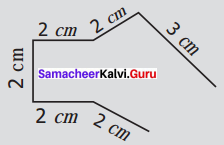Is the perimeter of the given shape possible ? why ?
Solution:
Not possible. Because perimeter is the length of the boundaries of any closed shape. It is not a closed shape.

Try These (Text book Page No.41)

Question 1.
Draw a shape with perimeter 16 cm in a dot sheet.
Solution: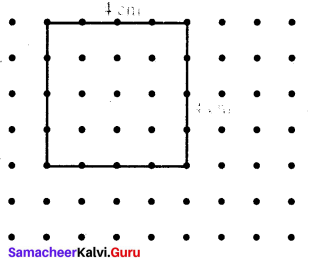Question 2.
What is the perimeter of a rectangle if the length is twice its breadth?
Solution:
Perimeter of a rectangle = 2(l + b) units
= 2 × (2b + b) units
= 2 × 3b units = 6b units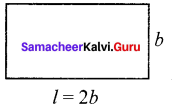Perimeter of a rectangle = 6b units.

Question 3.
What would be the perimeter of a square if its side is reduced to half?
Solution:
Let a side of a square = s units.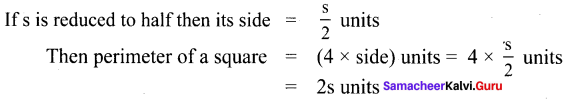Question 4.
What is the perimeter of a triangle if all sides are equal in length?
Solution:
Perimeter of a triangle P = sum of its 3 sides.
If all sides are equal, it is an equilateral triangle with side say a.
Perimeter = a + a + a units = 3a units

Activity (Text book Page No. 41)

Question 1.
Choose any five items like Table, A4 sheet, Note-book, etc in the classroom. Guess the approximate length of each side by observation and write down the estimated perimeter of the item. Then, measure by using ruler and record the actual perimeter and find the difference in the following table (to the nearest cm)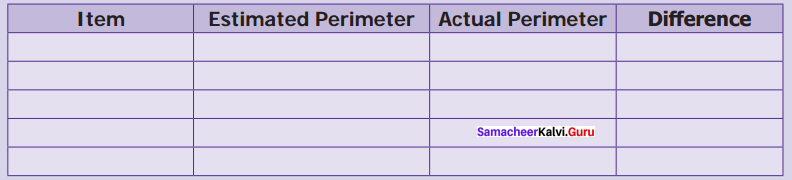Solution:
Activity to be done by the students themselvesTry this (Text book Page No. 42)

Question 1.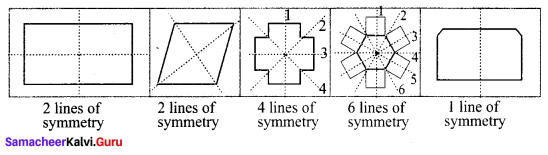Can different shapes have the same perimeter
Solution:
Yes, different shapes can have the same perimeter.

Try These (Text book Page No. 43)

Question 1.
Find the breadth of the rectangle with perimeter 14 m and length 4 m.
Solution:
Given perimeter of the rectangle P = 14 m
length l = 4 m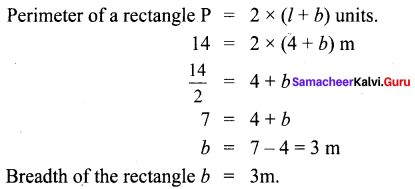Question 2.
The perimeter of an isosceles triangle is 21 cm. Find the measure of equal sides given that the third side is 5 cm.
Solution:
Perimeter of the triangle P = (a + b + c) units.
21 = [(a + b) + 5] cm
21 – 5 = a + b
16 = a + b
Here a and b are equal sides and let a = b
16 = a + a
2a = 16
$$a=\frac{16}{2}$$
a = 8 cm.
∴ Equal sides measure 8 cm.

Try these (Text book Page No. 44)

Question 1.
Find the number of tiles required to fill the area of following figures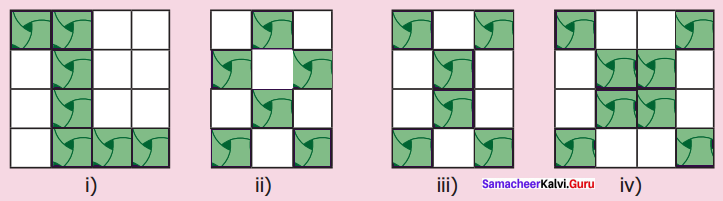Solution:
i) Total number of tiles required = 16
Number of tiles already filled = 7
Remaining required tiles = 16 – 7 = 9
ii) Total number of tiles required = 12
Number of tiles already filled = 6
Remaining required tiles = 12 – 6 = 6
iii) Total number of tiles required = 12
Number of tiles already filled = 6
Remaining required tiles = 12 – 6 = 6
iv) Total number of tiles required = 16
Number of tiles already filled = 8
Remaining required tiles = 16 – 8 = 8

Activity (Text book Page No. 45)

Question 1.
Mark the base and height of the following right angled triangle.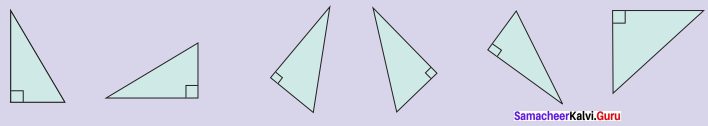Solution:
Activity to be done by the students themselves

Try These (Text book Page No. 45)

Question 1.
Draw the following in a graph sheet?
i) Two different rectangles whose areas are 16cm2
Solution: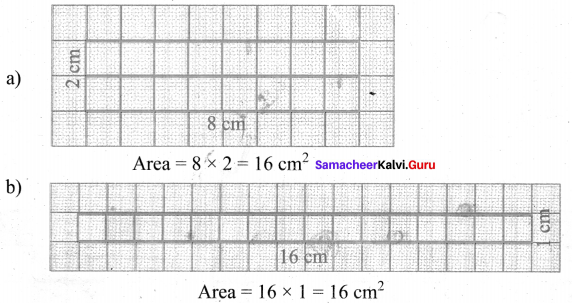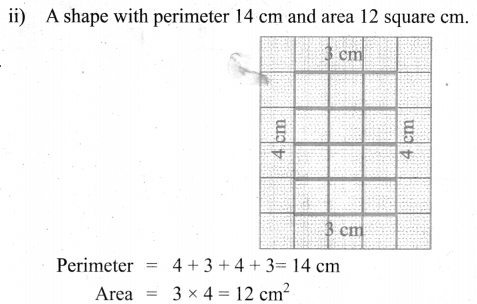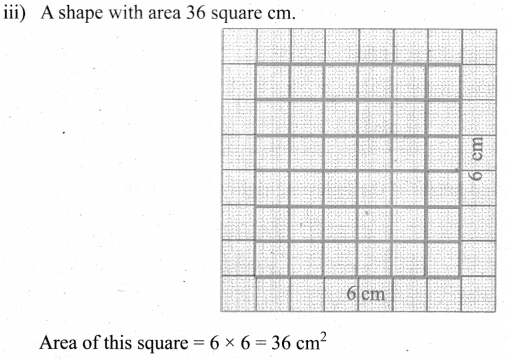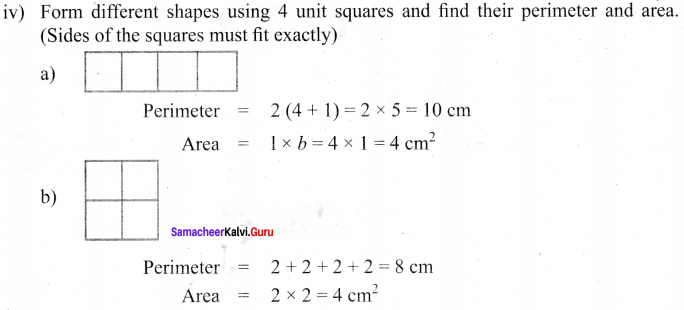Activity (Text book Page No. 46)

Question 1.
Find the area of the given ‘L’ shaped rectangular figure by dividing it into squares of equal sizes
Solution:
Activity to be done by the students themselves

Try this (Text book Page No.46)Question 2.
Can you find the area of ‘L’ shaped figure as the difference between two areas.
Solution:
Here Area of L shape = (Area of the rectangle ABCD)
(Area of the rectangle GFHB) = (2 × 3) – (2 × 1) cm2
= 6 – 2 cm2 = 4 cm2

Try these (Text book Page No. 46)

Question 1.
Measure using ruler and find the perimeter of each of the following diagram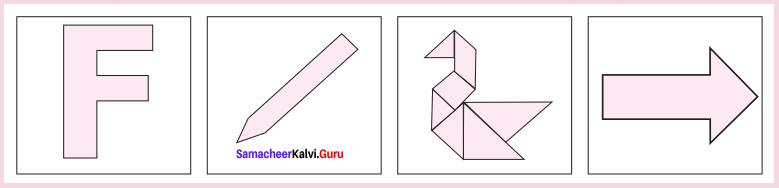Solution:
i) Perimeter = 2.5 + 0.5 + 1 + 1 + 0.5 + 1 + 0.5 + 1 + 0.5 + 1.5 = 10 cm
ii) Perimeter = 2.5 + 0.5 + 2.5 + 0.5 + 0.5 cm = 6.5 cm
iii) Perimeter = 0.5 + 1 + 0.5 + 0.5 + 1 + 1 + 0.5 + 1 + 1.5 + 0.5 + 1 + 0.5 cm = 9 cm
iv) Perimeter = 2 + 0.5 + 1.5 + 1.5 + 0.5 + 0.5 + 2 + 1 cm = 9.5 cm

Activity (Text book Page No 46)

Question 1.
Form all possible shapes of perimeter 80 cm with 9 identical squares, each of side 4 cm.
Solution:
Activity to be done by the students themselves

Activity

Question 2.
Cut a rectangular sheet along one of its diagonals. Two identical scalene right angled triangles are obtained. Join them along their sides of identical length in all possible ways. Six different shapes can be obtained. Four of them are given. Find the remaining two shapes. Find the perimeter of all the six shapes and fill in the table.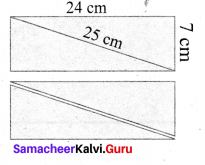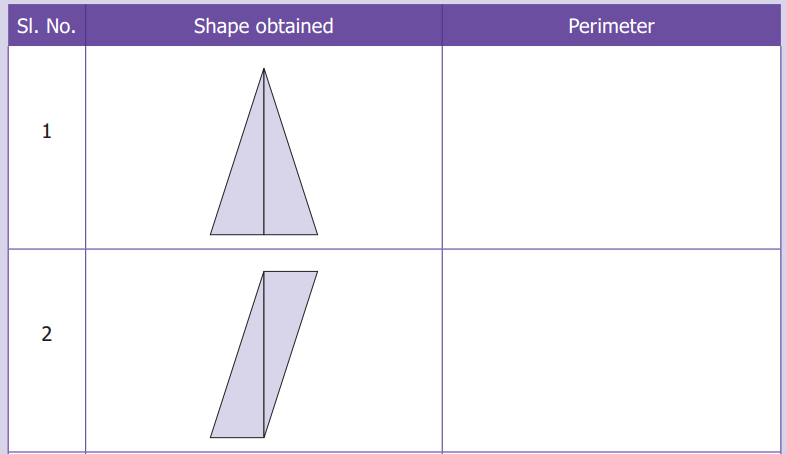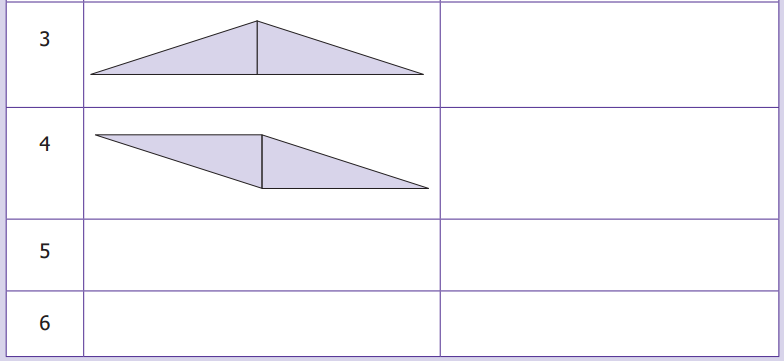Based on the above activity answer the following questions:
i) Are the perimeters same for all the shapes?
ii) Which shape has the longest perimeter?
iii) Which shape has the shortest perimeter?
iv) Are the areas of all the shapes same? why?
Solution:
Activity to be done by the students themselves

Try These (Text book Page No. 49)

Question 1.
Find the approximate area of the following figures: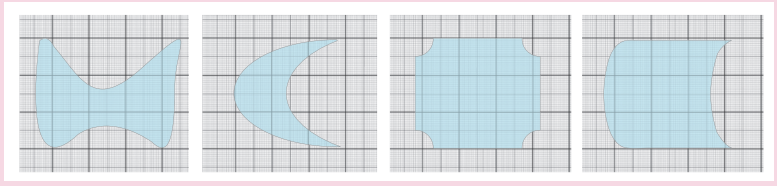Solution: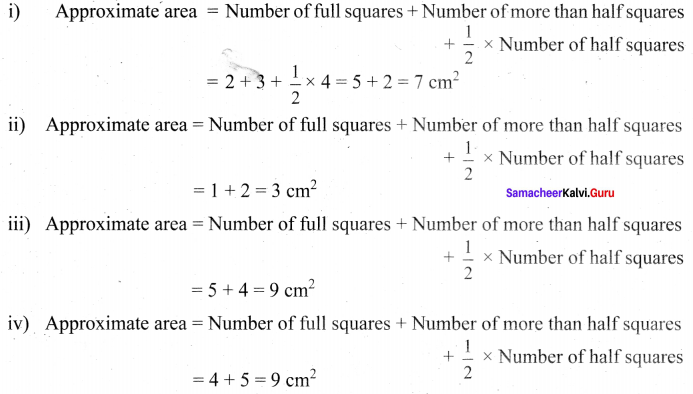Try these (Text book Page No. 50)

Question 2.
Fill in the blanks.
(i) 7 cm2 = ____ mm2
Hint: 1 cm2 = 100 m
Solution:
700

(ii) 10 m2 = ___ cm2
Hint: 1 m2 = 10000 cm2
Solution:
1,00,000

(iii) 3 km2 = ____ m2
Hint: 1 km2 = 1000000
Solution:
30,00,000